## Project MOSAIC migrates to ggformula

In 2017, Project MOSAIC announced `ggformula`, a new package that provides a formula interface to `ggplot2` graphics in R. (See, for example, ggformula: another option for teaching graphics in R to beginners.) This package provides a happy medium between `lattice` and `ggplot2` that allows beginners to “do powerful things quickly” by adopting the formula interface of `lattice` and R’s statistical modeling functions as a means to produce `ggplot2` graphics.

Over the past year, our experience with `ggformula` in our classes and in faculty development workshops together with the feedback we have received from other users have demonstrated `ggformula` to be flexible, yet easy to learn. As part of an ecosystem that emphasizes a formula interface of `lattice` and the core R statistical modeling functions early on and adds `tidyverse` concepts later, `ggformula` fits better with the rest of our toolkit than do either `lattice` or `ggplot2`, providing opportunities for more creativity with less volume.

The recent releases of several Project MOSAIC R packages (`mosaic`, `mosaicData`, `mosaicCore`, and `ggformula`) and the related `fastR2` package mark the official migration of Project MOSAIC from `lattice` to `ggformula` as its primary graphics system. Future development includes plans to release an updated version of `mosaicModel` which will interoperate with `ggformula` and a new package called `ggformulaExtra` (currently only available via Github) which adds additional functionality but relies on additional packages beyond `ggplot2`.

Many of the recent changes to the Project MOSAIC suite of packages will go largely unnoticed by most users but were necesary to allow `ggformula` to interoperate with the newest version of `ggplot2`. Among the small number of more noticeable changes are a change in `gf_smooth()` so that it no longer displays confidence bands by default (use `se = TRUE` to turn them on), expanded support for “rugs”, support for horizontal versions of histograms, boxplots, and violin plots (using the ggstance package), and the addition of `gf_sf()` for improved support for choropleth maps (based on the new `geom_sf()` in `ggplot2`). Along the way, we also did some light housekeeping (improving documentation, etc.) and migrated most of our package examples from `lattice` to `ggformula`.

The basic form of the formula interface is

``goal(y ~ x, data = myData)``
``````
``````
which corresponds to SAS code like

``PROC GOAL DATA = MYDATA; MODEL Y = X; RUN;``
``````
``````
`goal()` can be replaced by a graphing (e.g., `gf_point()`) or modeling (e.g., `lm()`) function with the number of variables involved in the formula varying with the complexity of the plot or model desired.

``````library(mosaic)              # load the mosaic package (and ggformula)
gf_point(length ~ width, data = KidsFeet)                  # scatter plot
lm(length ~ width, data = KidsFeet) %>% msummary()   # linear model``````
``````##             Estimate Std. Error t value Pr(>|t|)
## (Intercept)   9.8172     2.9381   3.341  0.00192 **
## width         1.6576     0.3262   5.081  1.1e-05 ***
##
## Residual standard error: 1.025 on 37 degrees of freedom
## Multiple R-squared:  0.411,  Adjusted R-squared:  0.3951
## F-statistic: 25.82 on 1 and 37 DF,  p-value: 1.097e-05``````
``````
``````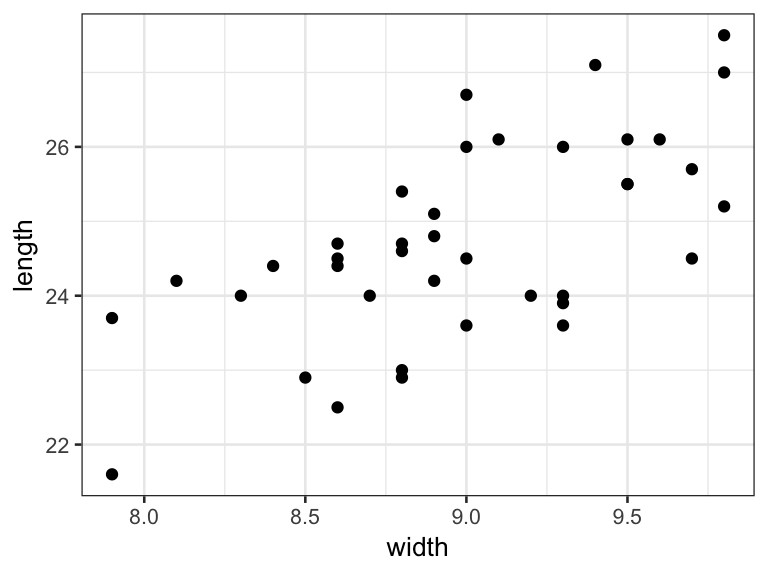Users of `lattice`-based Project MOSAIC materials should have little trouble migrating to `ggformula` since the types of plots that were easiest to construct with `lattice` can be created very similarly using `ggformula`. For example, the following two commands are essentially equivalent (although the resulting plots have a different appearence).

``````    histogram( ~ age | sex, data = HELPrct,    width = 2, col  = "navy")
gf_dhistogram( ~ age | sex, data = HELPrct, binwidth = 2, fill = "navy")``````
``````
``````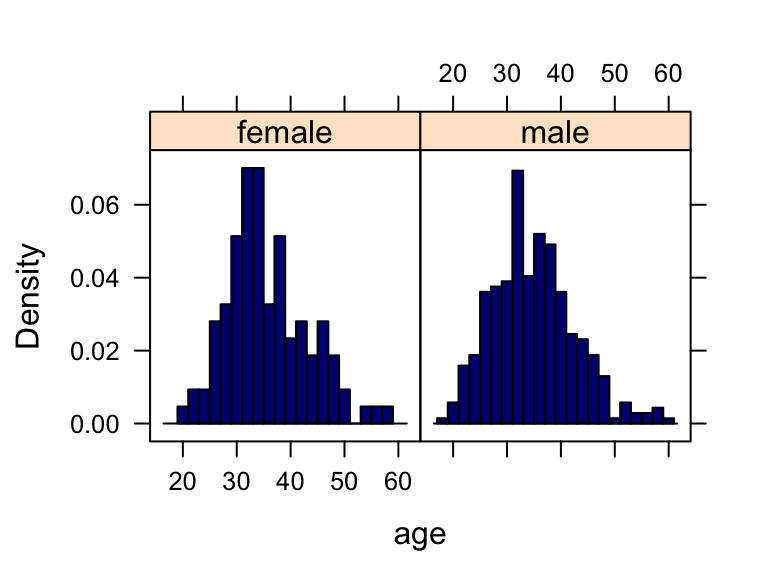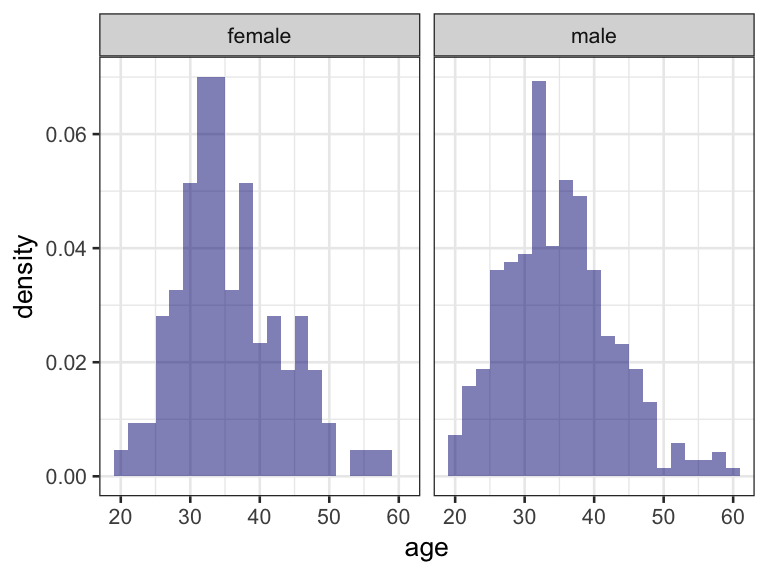It is much simpler, however, to create complex plots using `ggformula` because multiple layers can be stacked using the `maggrittr` pipe (`%>%`, which we often read as “then”) familiar to users of the `tidyverse` suite of packages (and many others as well).

``````gf_jitter(Sepal.Length ~ Sepal.Width, data = iris, color = ~ Species) %>%
gf_density2d(alpha = 0.4) %>%
gf_jitter(geom = "rug", alpha = 0.7) %>%
gf_lm(linetype = "dashed") %>%
gf_refine(scale_color_brewer(type = "qual"))``````
``````
``````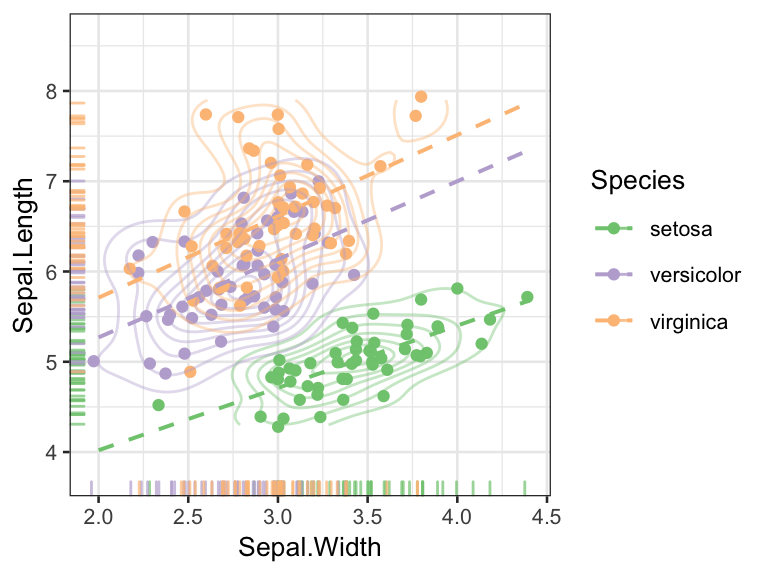As part of the migration to `ggformula`, a number of related resources have been or are being converted from `lattice` to `ggformula` as well. These include companion volumes for several popular statistics text books, our series of “Little Books”, the Minimal R Vignette, and a side-by-side comparison of `lattice` and `ggformula`. In addition, the second edition of Foundations and Applications of Statistics (Pruim, 2018) uses `ggformula` throughout.

An eventual migration from `ggformula` to native `ggplot2`, while not strictly necessary (since the same plots can be made in either system), is easier than the migration from `lattice` since the underlying grammar and much of the nomenclature of `ggformula` is borrowed from `ggplot2`. In the meantime, equivalent `ggformula` code is generally less verbose and simpler for novices to understand and produce. And the use of `%>%` for layering avoids the errors that creap in when moving between `tidyverse`, which also uses `%>%`, and `ggplot2` which uses `+`. Indeed, data flows can be directed seamlessly into `ggformula` plotting commands. This can be useful as a debugging step when creating data pipelines or as a way to create a plot for which there is no need to save the pre-processed data.

``````Galton %>%
filter(sex == "M") %>%  # select only male adult children
group_by(family) %>%      #
sample_n(1) %>%           # choose only one male from each family
ungroup %>%               #
mutate(                     # compute z-scores for parents' heights
zfather = round(mosaic::zscore(father), 2),
zmother = round(mosaic::zscore(mother), 2)
) %>%
gf_jitter(zfather ~ zmother, alpha = 0.5,
title = "Standardized heights of parents",
caption = "Source: Galton") %>%
gf_lm()``````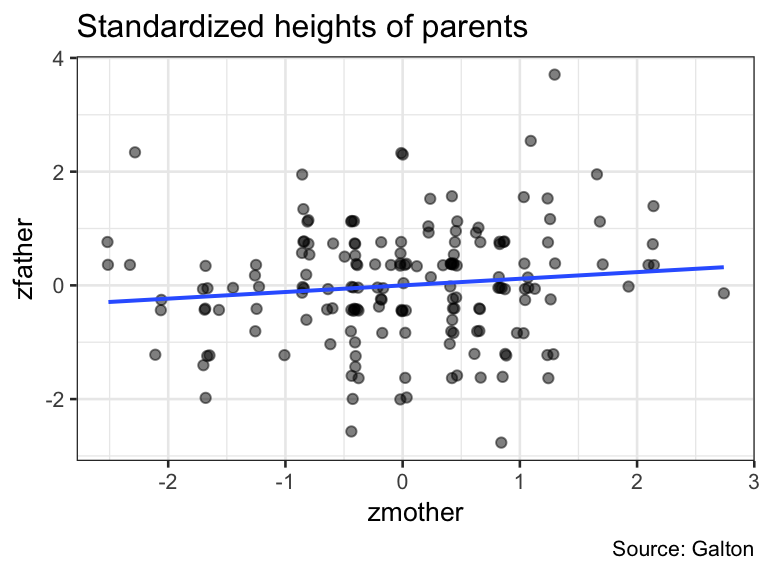It has been over a year since I have used either `lattice` or `ggplot2` for anything other than comparison examples. My co-authors and I have found the switch from `lattice` to `ggformula` to be both straightforward (for us) and advantageous (for our students). We encourage you to give it a try in your own work and with your students.